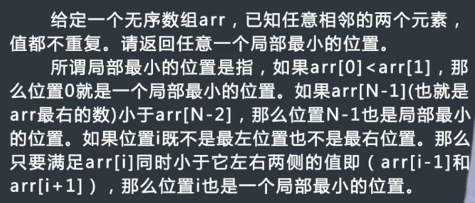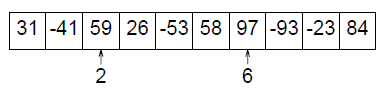# 题目一：

给定一个数组arr，求出需要排序的最短子数组长度

时间o(n),空间o(1)

有序的数组中，任意一个数字，一定小于左边的数大于右边的数。

我们找到的需要排序的子数组，显然是比右边最小的值大，或比左边最大的值小。

我们初始化变量noMinindex=-1;从右往左遍历，记录经过的最小值为min，若当前数大于min，说明，如果要有序，min一定要放      在当前数左边，我们更新noMinindex。

也就是说，我们的noMinindex是负责记录最左边出现这种情况的位置。我们反方向处理出noMaxindex

他们组成的区间就是最短需要排序的部分了

public class MinLengthForSort {
public static int getMinLength(int[] arr) {
if (arr == null || arr.length < 2) {
return 0;
}
int min = arr[arr.length - 1];
int noMinIndex = -1;
for (int i = arr.length - 2; i != -1; i--) {
if (arr[i] > min) {
noMinIndex = i;
} else {
min = Math.min(min, arr[i]);
}
}
if (noMinIndex == -1) {
return 0;
}
int max = arr;
int noMaxIndex = -1;
for (int i = 1; i != arr.length; i++) {
if (arr[i] < max) {
noMaxIndex = i;
} else {
max = Math.max(max, arr[i]);
}
}
return noMaxIndex - noMinIndex + 1;
}
public static void main(String[] args) {
int[] arr = { 1, 2, 4, 7, 10, 11, 7, 12, 6, 7, 16, 18, 19 };
System.out.println(getMinLength(arr));

}

}


# 题目二：

给定一个数组，找出出现次数超过一半的数字

DP：扫一遍一个变量count记录解出现的次数，是当前解就++，否则--，count为负就换掉当前解。（解释：想象解全都挨在         一起（前面），count先达到最大，然后减为1或0，而其他数字先出现，可能会使正确解的count减为负数，但都会使正确解        在后面更多，从而保证了结束时肯定为正确解）

int main()
{
int n;//个数
scanf("%d",&n);
int temp,k,count=0;
while(n--)
{
scanf("%d",&temp);
if(temp==k)count++;
else
{
count--;
if(count<0){count=0;k=temp;}
}
}
printf("%d\n",k);
}

# 题目三：

0       1       2       5

2       3       4       7

4       4       4       8

5       7       7       9

1.从矩阵最右上角的数开始寻找（row=0,col=M-1）。

2.比较当前数matrix[row][col]与K的关系：

3.如果找到越界都没有发现与K相等的数，则返回false。

/**
* 在行列都排好序的矩阵中找数
*/
public class IsContains {
public boolean isContains(int[][] matrix, int K) {
int row = 0;
int col = matrix.length - 1;
while (row < matrix.length && col > -1) {
if (matrix[row][col] == K) {
return true;
} else if (matrix[row][col] > K) {
col--;
} else {
row++;
}
}
return false;
}
}

# 题目四：转圈打印矩阵

思路：

1          2          3          4

5                                  8

9                                 12

13       14        15        16

6        7

10      11

/**
* 转圈打印矩阵
*/
public class PrintMatrixSpiralOrder {
public void spiralOrderPrint(int[][] matrix) {
int tR = 0;
int tC = 0;
int dR = matrix.length - 1;
int dC = matrix.length - 1;
while (tR <= dR && tC <= dC) {
printEdge(matrix, tR++, tC++, dR--, dC--);
}
}

//转圈打印一个子矩阵的外层，左上角点（tR,tC），右下角点（dR,dC）
private void printEdge(int[][] matrix, int tR, int tC, int dR, int dC) {
if (tR == dR) {//子矩阵只有一行时
for (int i = tC; i <= dC; i++) {
System.out.print(matrix[tR][i] + " ");
}
} else if (tC == dC) {//子矩阵只有一列时
for (int i = tR; i <= dR; i++) {
System.out.print(matrix[i][tC] + " ");
}
} else {//一般情况
int curCol = tC;
int curRow = tR;
while (curCol != dC) {//从左向右
System.out.print(matrix[tR][curCol] + " ");
curCol++;
}
while (curRow != dR) {//从上到下
System.out.print(matrix[curRow][dC] + " ");
curRow++;
}
while (curCol != tC) {//从右到左
System.out.print(matrix[dR][curCol] + " ");
curCol--;
}
while (curRow != tR) {//从下到上
System.out.print(matrix[curRow][tC] + " ");
curRow--;
}
}
}
}

# 题目五：将正方形矩阵顺时针转动90°

1        2        3        4

5        6        7        8

9        10      11      12

13      14      15      16

13      9       5      1

14      10     6      2

15      11     7      3

16      12     8      4

1          2          3          4

5                                  8

9                                 12

13       14        15        16

6       7

10     11

/**
* 将正方形矩阵顺时针旋转90°
*/
public class RotateMatrix {
public static void rotate(int[][] matrix) {
int tR = 0;
int tC = 0;
int dR = matrix.length - 1;
int dC = matrix.length - 1;
while (tR < dR) {
rotateEdge(matrix, tR++, tC++, dR--, dC--);
}
}

private static void rotateEdge(int[][] matrix, int tR, int tC, int dR, int dC) {
int times = dC - tC;//times就是总的组数
int temp = 0;
for (int i = 0; i != times; i++) {//一次循环就是一组占据调整
temp = matrix[tR][tC + i];
matrix[tR][tC + i] = matrix[dR - i][tC];
matrix[dR - i][tC] = matrix[dR][dC - i];
matrix[dR][dC - i] = matrix[tR - i][dC];
matrix[tR - i][dC] = temp;
}
}
}

# 题目六：“之”字形打印矩阵

1        2        3        4

5        6        7        8

9        10      11      12

“之”字形打印的结果为：1,2,5,9,6,3,4,7,10,11,8,12

/**
* 之字形打印矩阵
*/
public class PrintMatrixZigZag {
public static void printMatrixZigZag(int[][] matrix) {
int tR = 0;
int tC = 0;
int dR = 0;
int dC = 0;
int endRow = matrix.length - 1;
int endCol = matrix.length - 1;
boolean fromUp = false;
while (tR != endRow + 1) {
printLevel(matrix, tR, tC, dR, dC, fromUp);
tR = tC == endCol ? tR + 1 : tR;
tC = tC == endCol ? tC : tC + 1;
dR = dR == endRow ? dR : dR + 1;
dC = dR == endRow ? dC + 1 : dC;
fromUp = !fromUp;
}
System.out.println();
}

private static void printLevel(int[][] matrix, int tR, int tC, int dR, int dC, boolean fromUp) {
if (fromUp) {
while (tR != dR + 1) {//从左下到右上
System.out.print(matrix[tR++][tC--] + " ");
}
} else {
while (dR != tR - 1) {//从右上到左下
System.out.print(matrix[dR--][dC++] + " ");
}
}
}
}

# 题目七

public static void sort1(int[] arr) {
int tmp = 0;
int next = 0;
for (int i = 0; i != arr.length; i++) {
tmp = arr[i];
while (arr[i] != i + 1) {
next = arr[tmp - 1];
arr[tmp - 1] = tmp;
tmp = next;
}
}
}

# 题目八考虑最左和最右的元素：如果arr<arr  return 0; arr[N-1]<arr[N-2] return N-1;

考虑最中间元素，如果中间元素大于它左边的元素，那么局部最小值就应该在数组的左半部分

如果中间元素小于大于它右边的元素，那么局部最小值就应该在数组的右半部分

中间元素既小于它左边的值又小于它右边的值，那么它就是局部最小

# 题目九

	public int[] product1(int[] arr) {
if(arr==null || arr.length<2) {
return null;
}
int count=0;//0的个数
int all=1;//除0以外的数的乘积
for(int i=0;i!=arr.length;i++) {
if(arr[i]!=0) {
all*=arr[i];
}else {
count++;
}
}
int[] res=new int[arr.length];
if(count==0) {
for(int i=0;i!=arr.length;i++) {
res[i]=all/res[i];
}
}else if(count==1) {
for(int i=0;i!=arr.length;i++) {
if(arr[i]==0) {
res[i]=all;
}
}
}
return res;
}

# 题目十：子数组的最大累加和问题1）

for(i = 1; i <= n; i++)
scanf("%d", &num[i]);
ans = num;
for(i = 1; i <= n; i++)
{
for(j = i; j <= n; j++)
{
s = 0;
for(k = i; k <= j; k++)
s += num[k];
if(s > ans)
ans = s;
}
}

2）

for(i = 1; i <= n; i++)
scanf("%d", &num[i]);
sum = 0;
for(i = 1; i <= n; i++) {
sum[i] = num[i] + sum[i - 1];
}
ans = num;
for(i = 1; i <= n; i++) {
for(j = i; j <= n; j++) {
s = sum[j] - sum[i - 1];
if(s > ans) ans = s;
}
}

3）

int solve(int left, int right)
{
if(left == right)
return num[left];

mid = (left + right) / 2;
lans = solve(left, mid);
rans = solve(mid + 1, right);

sum = 0, lmax = num[mid], rmax = num[mid + 1];
for(i = mid; i >= left; i--) {
sum += num[i];
if(sum > lmax) lmax = sum;
}
sum = 0;
for(i = mid + 1; i <= right; i++) {
sum += num[i];
if(sum > rmax) rmax = sum;
}

ans = lmax + rmax;
if(lans > ans) ans = lans;
if(rans > ans) ans = rans;
return ans;
}

int main(void)
{
scanf("%d", &n);
for(i = 1; i <= n; i++)
scanf("%d", &num[i]);

printf("%d\n", solve(1, n));
return 0;
}

4）动态规划入门思想

for(i = 1; i <= n; i++)
scanf("%d", &num[i]);

num = 0;
ans = num;
for(i = 1; i <= n; i++)
{
if(num[i - 1] > 0)
num[i] += num[i - 1];
else
num[i] += 0;
if(num[i] > ans)
ans = num[i];
}

# 题目十一、子矩阵的最大累加和问题

1   2  3   4

-1 -2  1   2

1   3   -2  1

-1  -2  -1  -3

1  2  3  4

0  0  4  6

1  3  2  7

0  1  1  4

0-1，1-2，1-3，4-4=-1，-1，-2，0就是1-3行压缩后的结果

public class SubMatrixMaxSum {
public static int maxSum(int[][] m) {
if (m == null || m.length == 0 || m.length == 0) {
return 0;
}
int max = Integer.MIN_VALUE;
int cur = 0;
int[] s = null; // 累加数组
for (int i = 0; i != m.length; i++) {
s = new int[m.length];
for (int j = i; j != m.length; j++) {
cur = 0;
for (int k = 0; k != s.length; k++) {
s[k] += m[j][k];
cur += s[k];
max = Math.max(max, cur);
cur = cur < 0 ? 0 : cur;
}
}
}
return max;
}

public static void main(String[] args) {
int[][] matrix = { { -90, 48, 78 }, { 64, -40, 64 }, { -81, -7, 66 } };
System.out.println(maxSum(matrix));

}

}


# 题目十二、子数组的最大累乘积

## 思路：

• max*arr[i]//本身乘之前的最大累乘
• min*arr[i]//可能是负负得正变成最大的
• arr[i]//可能就是它本身，比如之前的max小于1

public class SubArrayMaxProduct {

public static double maxProduct(double[] arr) {
if (arr == null || arr.length == 0) {
return 0;
}
double max = arr;
double min = arr;
double res = arr;
double maxEnd = 0;
double minEnd = 0;
for (int i = 1; i < arr.length; ++i) {
maxEnd = max * arr[i];
minEnd = min * arr[i];
max = Math.max(Math.max(maxEnd, minEnd), arr[i]);
min = Math.min(Math.min(maxEnd, minEnd), arr[i]);
res = Math.max(res, max);
}
return res;
}

public static void main(String[] args) {
double[] arr = { -2.5, 4, 0, 3, 0.5, 8, -1 };
System.out.println(maxProduct(arr));

}

}


# 题目十三：调整有序的arr数组，使得左半部分有序且不重复，不用保证右边是否有序。

u : 左边的最后位置，即0---u为答案
i : 从u到右遍历
当arr[i]和arr[u]不相等时，说明是目前遇到的最大的数，此时调换arr[u+1]和arr[i]

	public static void leftUnique(int[] arr) {
if (arr == null || arr.length < 2) {
return;
}
int u = 0;
int i = 1;
while (i != arr.length) {
if (arr[i++] != arr[u]) {
swap(arr, ++u, i - 1);
}
}
}
	public static void swap(int[] arr, int index1, int index2) {
int tmp = arr[index1];
arr[index1] = arr[index2];
arr[index2] = tmp;
}

# 题目十四：数组arr中只有三种值：0，1，2，请排序

	public static void sort(int[] arr) {
if (arr == null || arr.length < 2) {
return;
}
int left = -1;
int index = 0;
int right = arr.length;
while (index < right) {
if (arr[index] == 0) {
swap(arr, ++left, index++);
} else if (arr[index] == 2) {
swap(arr, index, --right);
} else {
index++;
}
}
}

# 题目十五：数组arr，给定k，请实现比k小的放左边，等于k的放中间，大于k的放右边

03-0510602-0771
11-19250
05-192万+
05-2654
12-1087
09-2572
08-171598
09-13106
09-19179
03-301120
12-241万+
04-291万+
02-0774
08-14447
©️2020 CSDN 皮肤主题: 书香水墨 设计师:CSDN官方博客